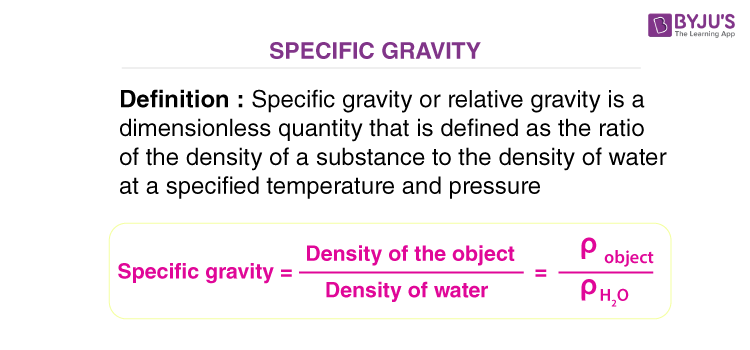# Specific Gravity

Have you wondered why some objects sink while others float? Many factors come into play in determining if an object will sink or float, and specific gravity is one of the main deciding factors. In this article, let us understand what is specific gravity in detail.

## What Is Specific Gravity?

Specific Gravity or relative gravity is a dimensionless quantity that is defined as the ratio of the density of a substance to the density of the water at a specified temperature and is expressed as

$$\begin{array}{l}SG=\frac{\rho _{substance}}{\rho _{H_2O}}\end{array}$$

It is common to use the density of water at 4 oC as a reference point as water at this point has the highest density of 1000 kg/m3.Given below is a table that lists the value of specific gravity for some common materials.

 Material Specific Gravity Dry Air 0.0013 Alcohol 0.82 Carbon dioxide 0.00126 Cast Iron 7.20 Petrol 0.72 Rubber 0.96 Wood Oak 0.77 Nylon 1.12

### Watch the video to understand the concept of specific gravity with the help of an example.## Calculating Specific Gravity

Specific gravity is determined by dividing the density of a material by the density of water at 4 degrees Celsius. For the calculation, the density of the material and that of the water must be expressed in the same units.

### Density Of Water In Various Units

• 1000 kilograms per cubic meter
• 1 gram per cubic centimeter
• 62.43 pounds per cubic foot
• 0.036 pounds per cubic inch

### Solved Examples of Specific Gravity

#### Example 1: Calculate the specific gravity of iron.

The density of iron is 7850 kg/m3. The specific gravity of iron-related to water is calculated as follows:

$$\begin{array}{l}SG_{iron}=\frac{7850\,kg/m^3}{1000\,kg/m^3}=7.85\end{array}$$

Hence, the specific gravity of iron is 7.85.

#### Example 2: If the density of gold is 19300 kg/m3, what is the specific gravity of gold?

We can calculate the specific gravity of gold as follows:

$$\begin{array}{l}SG_{gold}=\frac{19300\,kg/m^3}{1000\,kg/m^3}=19.3\end{array}$$

Hence, the specific gravity of iron is 19.3.

#### Example 3: If the specific gravity of ice is 0.92, then what is the density of ice?

The density of ice can be calculated by interchanging the specific gravity formula as follows:

$$\begin{array}{l}SG=\frac{\rho _{substance}}{\rho _{H_2O}}\Rightarrow \rho _{substance}=SG\cdot \rho _{H_2O}\end{array}$$

Substituting the values in the above equation, we get

$$\begin{array}{l}\rho _{substance}=0.92\cdot 1000\,kg/m^3 =920\,kg/m^3\end{array}$$

## Specific Gravity of Gas

For gases, the specific gravity is normally calculated with reference to air. Specific gravity for gases is defined as the ratio of the density of the gas to the density of air at a specified temperature and pressure. The density of air at room temperature is 1.20 kg/m3.

$$\begin{array}{l}SG=\frac{\rho _{gas}}{\rho _{air}}\end{array}$$

In the exam, if the reference substance is not specified for a specific gravity problem, it is usually safe to assume that you are using this as your reference substance.

## Factors Affecting Specific Gravity

As discussed, specific gravity is the measure of the density of an object in comparison to the density of water at an exact temperature and pressure. Changes in the pressure and temperature affect the density of objects and liquids, thereby affecting the specific gravity of objects and liquids. Standard temperature and pressure are used in the calculation of specific gravity. If failed to regulate the outside influences, then the specific gravity will change.

## Applications of Specific Gravity

Specific gravity has a wide range of applications, following are a few of the applications:

• The gem purity can be determined by comparing its specific gravity with the already measured high purity level of another gem. This allows the gem’s value to be determined very quickly.
• Specific gravity is used by mineralogists and geologists to determine the mineral content of the rock.
• Urinary specific gravity is a measure of the concentration of solutes in the urine. The test is a routine part of a urinalysis.

For better understanding, you might want to check out the following articles:

## Frequently Asked Questions on Specific Gravity

Q1

### What does Specific Gravity tell us?

The specific gravity of an object can tell us based on its value if the object will sink or float in our reference substance.

Q2

### What is the difference between specific gravity and specific weight?

Specific weight is the ratio of the weight of an object to the object’s volume. In the case of specific gravity, it is the ratio of an object’s density to the density of water.

Q3

### Which metal has the highest specific gravity?

Lead has the highest specific gravity.

Q4

### Which metal has the lowest specific gravity?

Lithium has the lowest specific gravity.

Q5

### Which instrument measures the specific gravity of liquids?

A hydrometer is used to measure the specific gravity of liquids.

Q6

### What is a urine specific gravity test?

Urine specific gravity test compares the density of urine with the density of water. The test gives information about how well the kidneys concentrate the urine and a person’s hydration status.

Q7

### What is specific gravity in a gemstone?

The specific gravity in a gemstone is the ratio of the density of the gemstone to the density of water.

Q8

### Which is the temperature at which water has the highest density?

4 °C is the temperature at which water has the highest density.

Q9

### What is the specific gravity of rubber?

The specific gravity of rubber is 0.96 kg/m3.

Q10

### What is the main application of specific gravity?

The concept of specific gravity is utilised by geologists and mineralogists to analyse the mineral constituents of rocks.

Stay Tuned to BYJU’S and Keep Falling in Love with Learning!

Test Your Knowledge On Specific Gravity!